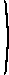# Mathematical methods for economic theory

Martin J. Osborne

## 6.2 Optimization with equality constraints: n variables, m constraints

The Lagrangean method can easily be generalized to a problem of the form
maxx f(x) subject to gj(x) = cj for j = 1, ..., m
with n variables and m constraints (where x = (x1, ..., xn)).

The Lagrangean for this problem is

L(x) = f(x) − ∑m
j=1
λj(gj(x) − cj).
That is, there is one Lagrange multiplier for each constraint.

As in the case of a problem with two variables and one constraint, the first-order condition is that x* be a stationary point of the Lagrangean. The “nondegeneracy” condition in the two variable case—namely that at least one of g'1(x1x2) and g'2(x1x2) is nonzero—is less straightforward to generalize. The appropriate generalization involves the Jacobian matrix of the constraint functions (g1, ..., gm), named in honor of Carl Gustav Jacob Jacobi (1804–1851) and defined as follows.

Definition
For j = 1, ..., m let gj be a differentiable function of n variables. The Jacobian matrix of (g1, ..., gm) at the point x is(∂g1/∂x1)(x) ... (∂g1/∂xn)(x)... ... ... (∂gm/∂x1)(x) ... (∂gm/∂xn)(x)
.
Proposition (Necessary conditions for an extremum)
Let f and gj for j = 1, ..., m be continuously differentiable functions of n variables defined on the set S, with m ≤ n, let cj for j = 1, ..., m be numbers, and suppose that x* is an interior point of S that solves the problem
maxx f(x) subject to gj(x) = cj for j = 1, ..., m.
or the problem
minx f(x) subject to gj(x) = cj for j = 1, ..., m.
or is a local maximizer or minimizer of f(x) subject to gj(x) = cj for j = 1, ..., m. Suppose also that the rank of the Jacobian matrix of (g1, ..., gm) at the point x* is m.

Then there exist unique numbers λ1, ..., λm such that x* is a stationary point of the Lagrangean function L defined by

L(x) = f(x) − ∑m
j=1
λj(gj(x) − cj).
That is, x* satisfies the first-order conditions
 L'i(x*) = fi'(x*) − ∑mj=1λj(∂gj/∂xi)(x*) = 0 for i = 1, ..., n.
In addition, gj(x*) = cj for j = 1, ..., m.
Source
For proofs, see Sydsæter (1981), Theorem 5.20 (p. 275) and Simon and Blume (1994), pp. 478–480. (Only Sydsæter argues explicitly that the Lagrange multipliers are unique.)
As in the case of a problem with two variables and one constraint, the first-order conditions and the constraint are sufficient for a maximum if the Lagrangean is concave, and are sufficient for a minimum if the Lagrangean is convex, as stated precisely in the following result. The proof is the same as the proof for the result for two variables and a single constraint.
Proposition (Conditions under which necessary conditions for an extremum are sufficient)
Let f and gj for j = 1, ..., m be continuously differentiable functions of n variables defined on the open convex set S. Let x* be an interior point of S that is a stationary point of the Lagrangean
L(x) = f(x) − ∑m
j=1
λ*j (gj(x) − cj).
Suppose further that gj(x*) = cj for j = 1, ..., m. Then
• if L is concave—in particular if f is concave and λ*jgj is convex for j = 1, ..., m—then x* solves the constrained maximization problem
• if L is convex—in particular if f is convex and λ*jgj is concave for j = 1, ..., m—then x* solves the constrained minimization problem
Example
Consider the problem
minx,y,z x2 + y2 + z2 subject to x + 2y + z = 1 and 2x − y − 3z = 4.
The Lagrangean is
 L(x, y, z) = x2 + y2 + z2 − λ1(x + 2y + z − 1) − λ2(2x − y − 3z − 4).
This function is convex for any values of λ1 and λ2, so that any interior stationary point is a solution of the problem. Further, the rank of the Jacobian matrix is 2 (a fact you can take as given), so any solution of the problem is a stationary point. Thus the set of solutions of the problem coincides with the set of stationary points.

The first-order conditions are

 2x − λ1 − 2 λ2 = 0 2y − 2 λ1 + λ2 = 0 2z − λ1 + 3 λ2 = 0
and the constraints are
 x + 2 y + z = 1 2 x − y − 3 z = 4
Solve first two first-order conditions for λ1 and λ2 to give
 λ1 = (2/5)x + (4/5)y λ2 = (4/5)x − (2/5)y.
Now substitute into last the first-order condition and then use the two constraints to get
x = 16/15, y = 1/3, z = −11/15,
with λ1 = 52/75 and λ2 = 54/75.

We conclude that (xyz) = (16/15, 1/3, −11/15) is the unique solution of the problem.

### Economic interpretation of Lagrange multipliers

In the case of a problem with two variables and one constraint we saw that the Lagrange multiplier has an interesting economic interpretation. This interpretation generalizes to the case of a problem with n variables and m constraints.

Consider the problem

maxx f(x) subject to gj(x) = cj for j = 1, ..., m,
where x = (x1, ..., xn). Let x*(c) be the solution of this problem, where c is the vector (c1, ..., cm) and let
f*(c) = f(x*(c)).
Then we have
f*j'(c) = λj(c) for j = 1, ..., m,
where λj is the value of the Lagrange multiplier on the jth constraint at the solution of the problem.

That is:

the value of the Lagrange multiplier on the jth constraint at the solution of the problem is equal to the rate of change in the maximal value of the objective function as the jth constraint is relaxed.
If the jth constraint arises because of a limit on the amount of some resource, then we refer to λj(c) as the shadow price of the jth resource.